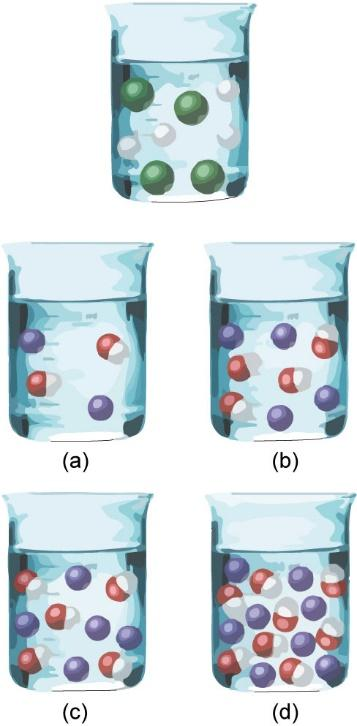×
Log in to StudySoup
Get Full Access to Introductory Chemistry - 5 Edition - Chapter 8 - Problem 100p
Join StudySoup for FREE
Get Full Access to Introductory Chemistry - 5 Edition - Chapter 8 - Problem 100p

Already have an account? Login here
×
Reset your password

# Solved: A hydrochloric acid solution will neutralize a sodium hydroxide solutionISBN: 9780321910295 34

## Solution for problem 100P Chapter 8

Introductory Chemistry | 5th Edition

• Textbook Solutions
• 2901 Step-by-step solutions solved by professors and subject experts
• Get 24/7 help from StudySoup virtual teaching assistantsIntroductory Chemistry | 5th Edition

4 5 1 283 Reviews
31
0
Problem 100P

PROBLEM 100P

A hydrochloric acid solution will neutralize a sodium hydroxide solution. Consider the molecular views showing one beaker of HCl and four beakers of NaOH. Which NaOH beaker will just neutralize the HCl beaker? Begin by writing a balanced chemical equation for the neutralization reaction.Step-by-Step Solution:

Problem 100P

A hydrochloric acid solution will neutralize a sodium hydroxide solution. Consider the molecular views showing one beaker of HCl and four beakers of NaOH. Which NaOH beaker will just neutralize the HCl beaker? Begin by writing a balanced chemical equation for the neutralization reaction.Step by step solution

Step 1 of 3

Neutralization reactions occur between an alkali and an acid. The identical equivalents of a particular acid and alkali get neutralized by each other. The products of such reactions are electrolyte and water.

Step 2 of 3

Step 3 of 3

##### ISBN: 9780321910295

This full solution covers the following key subjects: beaker, neutralize, solution, naoh, HCL. This expansive textbook survival guide covers 19 chapters, and 2046 solutions. Since the solution to 100P from 8 chapter was answered, more than 2417 students have viewed the full step-by-step answer. The answer to “A hydrochloric acid solution will neutralize a sodium hydroxide solution. Consider the molecular views showing one beaker of HCl and four beakers of NaOH. Which NaOH beaker will just neutralize the HCl beaker? Begin by writing a balanced chemical equation for the neutralization reaction.” is broken down into a number of easy to follow steps, and 44 words. The full step-by-step solution to problem: 100P from chapter: 8 was answered by , our top Chemistry solution expert on 05/06/17, 06:45PM. Introductory Chemistry was written by and is associated to the ISBN: 9780321910295. This textbook survival guide was created for the textbook: Introductory Chemistry, edition: 5.

Unlock Textbook Solution

Enter your email below to unlock your verified solution to:

Solved: A hydrochloric acid solution will neutralize a sodium hydroxide solution